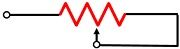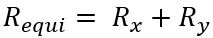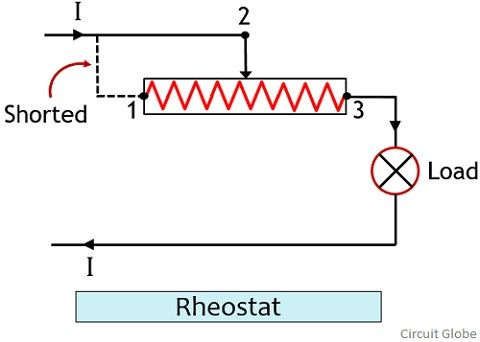# Difference Between Potentiometer and Rheostat

The crucial difference between potentiometer and rheostat is that a potentiometer is used to determine the unknown emf thus controls the circuit voltage. As against a rheostat is an instrument that controls the flow of current through a circuit.

The potentiometer measures the unknown emf or voltage by making a comparison with a known voltage. And the rheostat controls the flow of current through the circuit by varying the resistance. In this article we will discuss various factors that differentiates potentiometer from rheostat.

## Content: Potentiometer Vs Rheostat

### Comparison Chart

Basis for ComparisonPotentiometerRheostat
Instrument TypeSensorVariable resistor
SymbolNumber of terminals32
ControlsVoltageCurrent
Suitability of useFor low power applicationsFor high power applications
Composed ofMaterials like graphiteMaterials like metallic ribbon, carbon disks etc.
Type of connectionConnected in parallel with the circuit.Forms serial connection with the circuit.

### Definition of Potentiometer

An instrument that can determine the value of unknown emf in a circuit using the known emf is known as a potentiometer. It is a 3 terminal device and commonly known as POT. A potentiometer is commonly known to be device that controls the voltage across the circuit by varying the position of the wiper. A potentiometer does not require an external source of power for their operation.

While connecting a potentiometer in the circuit, it is kept in mind that both its terminals are connected to the circuit. Also, the wiper forms the connection with the circuit that provides the output voltage through that circuit. The value of this voltage will vary according the voltage levels of the two terminal points.

A potentiometer functions as a voltage divider whose output voltage shows variation according to the movement of the slider over the track.

The figure below shows the arrangement of a potentiometer:Basically the resistive element acts as two resistances placed in series and the position of the slider divides that resistance. So, by varying the position of the slider, the ratio of the two resistors shows variation.

The figure below shows the voltage divider circuit comprising of two resistors in series:The series combination of these two resistors will provide the equivalent resistor of the circuit asAs the two resistances are of two different values thus the voltage drop will also be different. The output voltage through the circuit will be given as:### Definition of Rheostat

A rheostat is a two terminal instrument that controls the current flowing through the circuit. We know that the current flowing through any circuit depends on the value of voltage and resistance. So, if the resistance of the circuit is varied then the current flowing through it also shows variation. This is the principle working of Rheostat.

A rheostat is a 2 terminal device whose one end is directly connected the circuit while the other is kept unconnected. However, like the potentiometer, here also the wiper terminal forms the connection with the circuit.

The figure below represents the arrangement of a rheostat:Sometimes the wiper terminal forms connection with the terminal 1 thereby short circuiting the region between terminal 1 and 2. The principle working of rheostat is such that, the resistance of the element will depend on its length through which the current is flowing.

Suppose if we move the slider in a position away from the connected terminal then in this case the length of the resistive region increases thus resistance will be high. Hence small amount of current to flow through it. While if the slider is moved towards the connected terminal then this resultantly reduces the resistive region length thereby reducing the resistance and hence the current flowing through it increases.

It is to be noteworthy here that despite changing the actual resistance, the movement of the slider is changing the length of the resistive region. This resultantly increases or decreases the resistance and therefore the current.

## Key Differences Between Potentiometer and Rheostat

1. A potentiometer is a 3 terminal instrument whose both the ends are connected with the circuit along with the wiper. While the rheostat is a two terminal device that forms connection with one end and the wiper or slider only.
2. A potentiometer controls the voltage of any circuit. However, a rheostat controls the current flowing through the circuit.
3. A potentiometer is mainly composed of graphite. Whereas rheostat is made up of materials like metal ribbon or carbon disks etc.
4. A potentiometer is a sensor while a rheostat is said to be variable resistor.
5. Potentiometer is mainly suitable for low power applications. As against a rheostat is mainly used in high power applications.
6. The potentiometer is connected in parallel with the circuit. While a rheostat forms serial connection with the circuit through which the flowing current is to be controlled.

### Conclusion

So, we can say that though both potentiometer and rheostat are the instruments that controls two different factors. But both utilizes the movement of slider or wiper in order to have significant change in the quantity required for their operation.